# 《Python有什么好学的》之修饰器

“Python有什么好学的”这句话可不是反问句，而是问句哦。

## 什么是修饰器，为什么叫修饰器

``````def hi():
"""hi func，假装是很复杂的函数"""
return 'hi'

def aop(func):
"""aop func"""
print('before func')
print(func())
print('after func')

if __name__ == '__main__':
aop(hi)
``````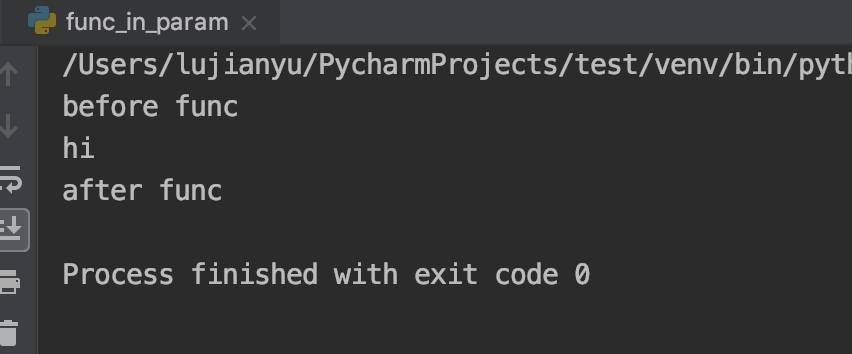1. 工作量大
2. 代码变得臃肿复杂
3. 原代码有多处调用了这些函数，可以会升级不完全

``````def aop(func):
"""aop func"""
def wrapper():
"""wrapper func"""
print('before func')
func()
print('after func')
return wrapper

@aop
def hi():
"""hi func"""
print('hi')

@aop
def hello():
"""hello func"""
print('hello')

if __name__ == '__main__':
hi()
hello()
``````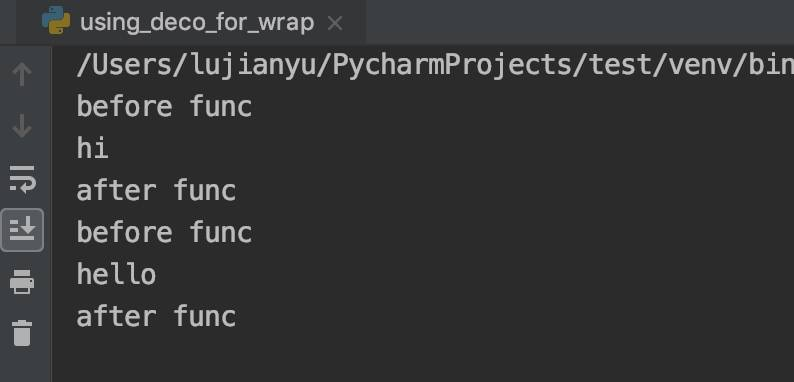## @是个什么

``````def hi():
"""hi func"""
print('hi')

def aop(func):
"""aop func"""
def wrapper():
"""wrapper func"""
print('before func')
func()
print('after func')
return wrapper

if __name__ == '__main__':
hi()

print('')

hi = aop(hi)
hi()
``````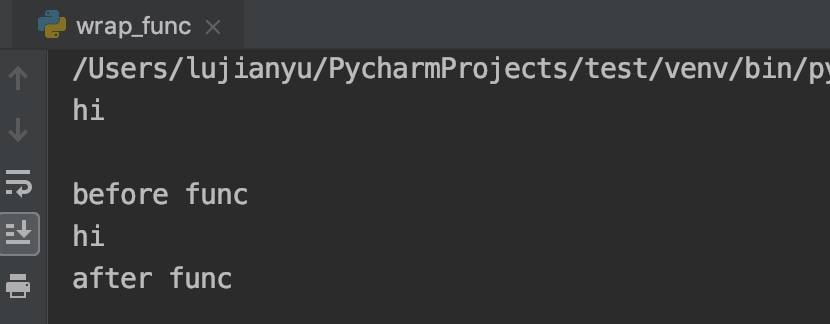`@aop` 等效于`hi = aop(hi)`

## 被调函数加参数

``````def aop(func):
"""aop func"""
def wrapper():
"""wrapper func"""
print('before func')
func()
print('after func')
return wrapper
``````

``````@aop
def hi_with_deco(a):
"""hi func"""
print('hi' + str(a))

if __name__ == '__main__':
# hi()
hi_with_deco(1)
``````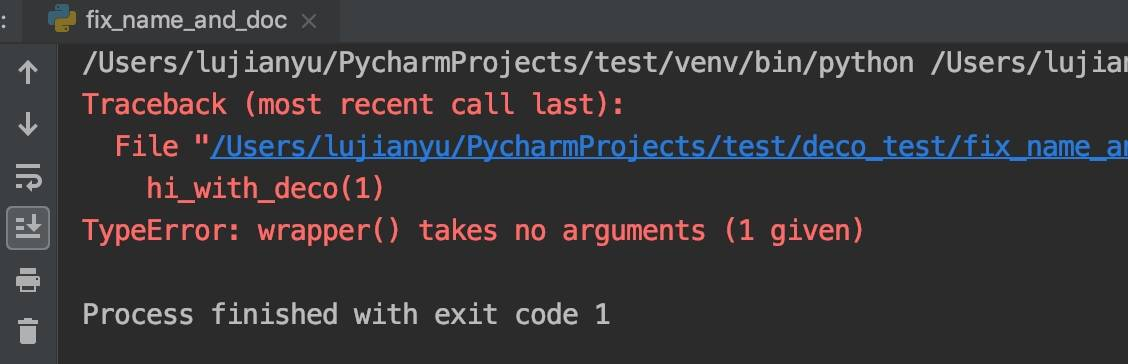``````from functools import wraps

def aop(func):
"""aop func"""
@wraps(func)
def wrap(*args, **kwargs):
print('before')
func(*args, **kwargs)
print('after')

return wrap

@aop
def hi(a, b, c):
"""hi func"""
print('test hi: %s, %s, %s' % (a, b, c))

@aop
def hello(a, b):
"""hello func"""
print('test hello: %s, %s' % (a, b))

if __name__ == '__main__':
hi(1, 2, 3)
hello('a', 'b')
``````## 带参数的修饰器

``````from functools import wraps

def aop_with_param(aop_test_str):
def aop(func):
"""aop func"""
@wraps(func)
def wrap(*args, **kwargs):
print('before ' + str(aop_test_str))
func(*args, **kwargs)
print('after ' + str(aop_test_str))
return wrap
return aop

@aop_with_param('abc')
def hi(a, b, c):
"""hi func"""
print('test hi: %s, %s, %s' % (a, b, c))

@aop_with_param('pppppp')
def hi2(a, b, c):
"""hi func"""
print('test hi: %s, %s, %s' % (a, b, c))

if __name__ == '__main__':
hi(1, 2, 3)
print('')
hi2(2, 3, 4)
``````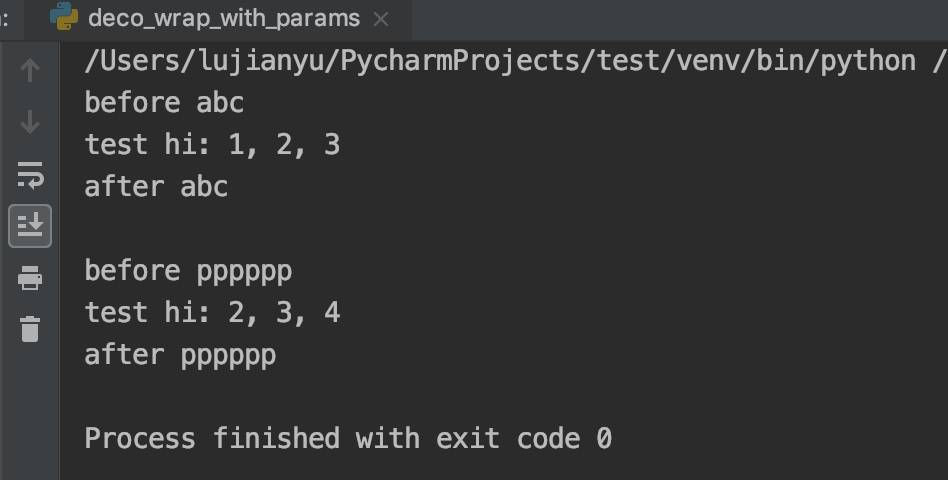## 修饰器类

``````from functools import wraps

class aop(object):
def __init__(self, aop_test_str):
self.aop_test_str = aop_test_str

def __call__(self, func):
@wraps(func)
def wrapper(*args, **kwargs):
print('before ' + self.aop_test_str)
func()
print('after ' + self.aop_test_str)

return wrapper

@aop('pppppp')
def hi():
print('hi')
``````

``````class sub_aop(aop):
def __init__(self, sub_aop_str, *args, **kwargs):
self.sub_aop_str = sub_aop_str
super(sub_aop, self).__init__(*args, **kwargs)

def __call__(self, func):
@wraps(func)
def wrapper(*args, **kwargs):
print('before ' + self.sub_aop_str)
super(sub_aop, self).__call__(func)()
print('after ' + self.sub_aop_str)
return wrapper

@sub_aop('ssssss', 'pppppp')
def hello():
print('hello')

if __name__ == '__main__':
hello()
``````

Categories: Python